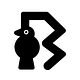# How Elixir helped to break the code challenge puzzle?

## Puzzle solving using elixir.

Elixir is fun when you find time to play with it.

I have broken into the first three room levels at breakthecode.tech challenges. So, With 6 distinct game rooms, Break The Code will put your logic, knowledge and creativity to the test. It is a set of challenging games with prizes galore, for you! [From Website]

In one of the rooms, I encountered a problem and took the elixir help to solve it. It is completely math. You can do with any programming language, but Elixir made it simple for me.

Let’s go…

# Problem

`A = 1 , B = 2A   A   *   A  A  =  A  B  A1   1   *   1  1  =  1  2  1A  A  * A  A  =  B  B  C  CFind A=? B=? C=?`

## Problem Analysis

I hope you alredy figured out what the problem is, if not here you go.

We need to find the three numbers such that it matches the given pattern.
The pattern is a multiplaction of a two similar digit number by itself, here A and it’s result will be the four digit number where first two numbers are of samekind, here B and last two numbers are samekind of other, here C.

`1 1 * 1 12 2 * 2 2 3 3 * 3 3...9 9 * 9 9`

Therefor the possible values of A, B, and C will be in the range `1..9`

## Number Prepartion

First step is we need to prepare a number of kind `A A`

`f(x) = (x*10)+xf(1) = (1*10) + 1 = (10) + 1 = 11f(2) = (2*10) + 2 = (20) + 2 = 22...f(9) = (9*10) + 9 = (90) + 9 = 99`

Then we need to multiply the prepare number to itself to give the result.

## Parsing Results

• Next thing is we need to filter the results which contains exactly 4 numbers. To do so we have to convert number to digits. In elixir we can achieve this with `Integer.digits/1` where it’s default base is 10.
• After converting to digits, we filter out whose digits count is equal to 4
• Next, we filter out whose first and second digits are of same kind and third and fourth digits are of another same kind

Ok! Talk is cheap, show me the code.

`#codethebreak.exsrange = 1..9prepare_num = fn x -> x * 10 + x endmultiply = fn {num, pre_num} -> {num, pre_num * pre_num} endto_digits = fn {num, mul_res} -> {num, Integer.digits(mul_res)} endlength_filter = fn {_num, digits} -> Enum.count(digits) == 4 endpattern_filter = fn {_num, digits} ->  [b, _, _, c] = digits  [b, b, c, c] == digitsendprint = fn {a, [b, _, _, c]} ->   """  A = #{a}, B=#{b}, C=#{c}  #{a} #{a} * #{a} #{a} = #{b} #{b} #{c} #{c}  """endrange|> Enum.map(&{&1, prepare_num.(&1)})|> Enum.map(multiply)|> Enum.map(to_digits)|> Enum.filter(length_filter)|> Enum.filter(pattern_filter)|> Enum.map(print)|> Enum.map(&IO.puts/1)`

The code is too explicit but self explains what it does.

Now it’s your time to play with Elixir.

# Happy Coding !!

Check out the GitHub repository on Killer Elixir Tips

Glad if you can contribute with a ★

Written by

Written by

## Blackode

#### coding, thoughts & ideas